Next: Off-axis Up: Flux of a Previous: Flux of a

#### On-axis,

the signal to noise ratio is given by: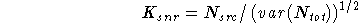where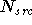is the number of source counts, and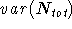is the variance in the total counts (source + background) and is typically given by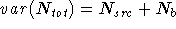, which assumes no uncertainty in the background.

The minimum detectable source count rate corresponding to a particular signal to noise ratio is given by the relationship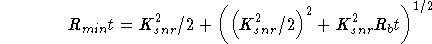where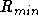denotes the minimum detectable source count rate,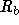the total background rate in the detection cell (about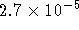counts s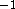), t the observation time, and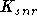the signal to noise ratio (snr) threshold for detection.

This is derived by combining the equations defining the snr and variance, and using the additional relation, counts = rate x time.

As an illustrative example, we show in Figure 11.3 in Appendix F, the point source sensitivity for= 5 versus observing time for a source with a power law spectrum. That is, we assume a point source with a power law spectrum of energy index 0.5 similar to the extragalactic X-ray background. A hydrogen column density of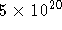cm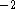is used. Notice that the sensitivity increases linearly with observing time for short observations where there are virtually no background counts in the detection cell. For observations that are long enough for background to be significant, longer than about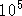s, the sensitivity increases only as the square root of the observation time.

In general, the conversion from HRI counts to energy flux depends on the spectrum of the X-ray source. For the power law spectrum characterized by the parameters given above, this conversion is given by: 1 count s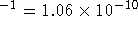erg cmsin the energy band 0.1 to 2.4 keV. The following section discusses the conversion from energy flux to count rate in more detail.

If you have problems/suggestions please send mail to rosat_svc@mpe-garching.mpg.de